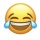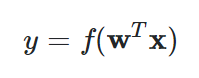Home
IT Knowledge
Inspiration
Languages
EN

# JavaScript - artificial neuron model

7 points
Created by:mkrieger1
516

In this short article, we would like to show an example of Artificial Neuron implementation in JavaScript.

Hint: the article describes McCulloch–Pitts (MCP) neuron that is commonly used in AI.

Quick solution:

``````// ONLINE-RUNNER:browser;

const activationFunction = (sum) => sum;

const inputs  = [ 1,   2,   3 ];  // x(1) x(2) x(3)
const bias    =   1;              // w(0)
const weights = [ 0,  0.5,  1 ];  // w(1) w(2) w(3)

const sum = weights.reduce((sum, weight, index) => sum + inputs[index] * weight, bias);
const output = activationFunction(sum);

console.log(output);  // 5``````

Where:

 `n` number of neuron inputs (number of neuron weights must be the same) `1` was placed on the above model only to show bias/threshold concept (multiplication by `1` is neutral but useful when we work on matrixes) `w(0)` weight called bias/threshold that is used to stimulate or suppress neuron activity `x(1)` - `x(n)` neuron input values (it is just an array of input values) `w(1)` - `w(n)` neuron weight values that are used to describe how strong influence has related input to the neuron `Σ` describes summation process `Σ = 1*w(0) + x(1)*w(1) + ... + x(n-1)*w(n-1) + x(n)*w(n)` `f(Σ)` describes sum passing through a neuron activation function (we can use different activation functions depending on the solved problem) `y` neuron output value

## Using matrix notation

The above model can be calculated using matrixes.Where:

• `w` is a column vector (array) of neuron weight values (from `w(0)` to `w(n)`),
• `x` is a column vector (array) of neuron input values (from `1` to `x(n)`),
• `f` is neuron activation function,
• `y` is neuron output value.

Note: go to the following articles to know more about vectors and matrixes:

## Simple JavaScript implementation

In this section, you can see simple step-by-step calculations made by artificial neurons.

``````// ONLINE-RUNNER:browser;

// Calculates sum using:
//    Σ = 1*w(0) + x(1)*w(1) + ... + x(n-1)*w(n-1) + x(n)*w(n)
//
const caclulateSum = (bias, weights, inputs) => {
let sum = bias;
for (let i = 0; i < weights.length; ++i) {
sum += inputs[i] * weights[i];
}
return sum;
};

// Used activation function
//
//   We use simple linear activation function (called Identity Function)
//   https://en.wikipedia.org/wiki/Activation_function
//
const activationFunction = (sum) => sum;

// Neuron calculation:

const inputs  = [ 1,   2,   3 ];  // x(1) x(2) x(3)

const bias    =   1;              // w(0)
const weights = [ 0,  0.5,  1 ];  // w(1) w(2) w(3)

const sum = caclulateSum(bias, weights, inputs);
const output = activationFunction(sum);

console.log(output);  // 5``````

## Reusable code example

In this section, you will find simple Neuron class implementation that can be combined into layers.

``````// ONLINE-RUNNER:browser;

function Neuron(bias, weights, activationFunction) {
this.bias = bias;
this.weights = weights;
this.calculate = function(inputs) {
var sum = this.bias;
for (var i = 0; i < this.weights.length; ++i) {
sum += inputs[i] * this.weights[i];
}
return activationFunction(sum);
};
}

// Usage example:

var identityFunction = function(sum) {
return sum;
};

var neuron = new Neuron(1,  [0, 0.5, 1], identityFunction);
var output = neuron.calculate([1, 2, 3]);

console.log(output);  // 5``````